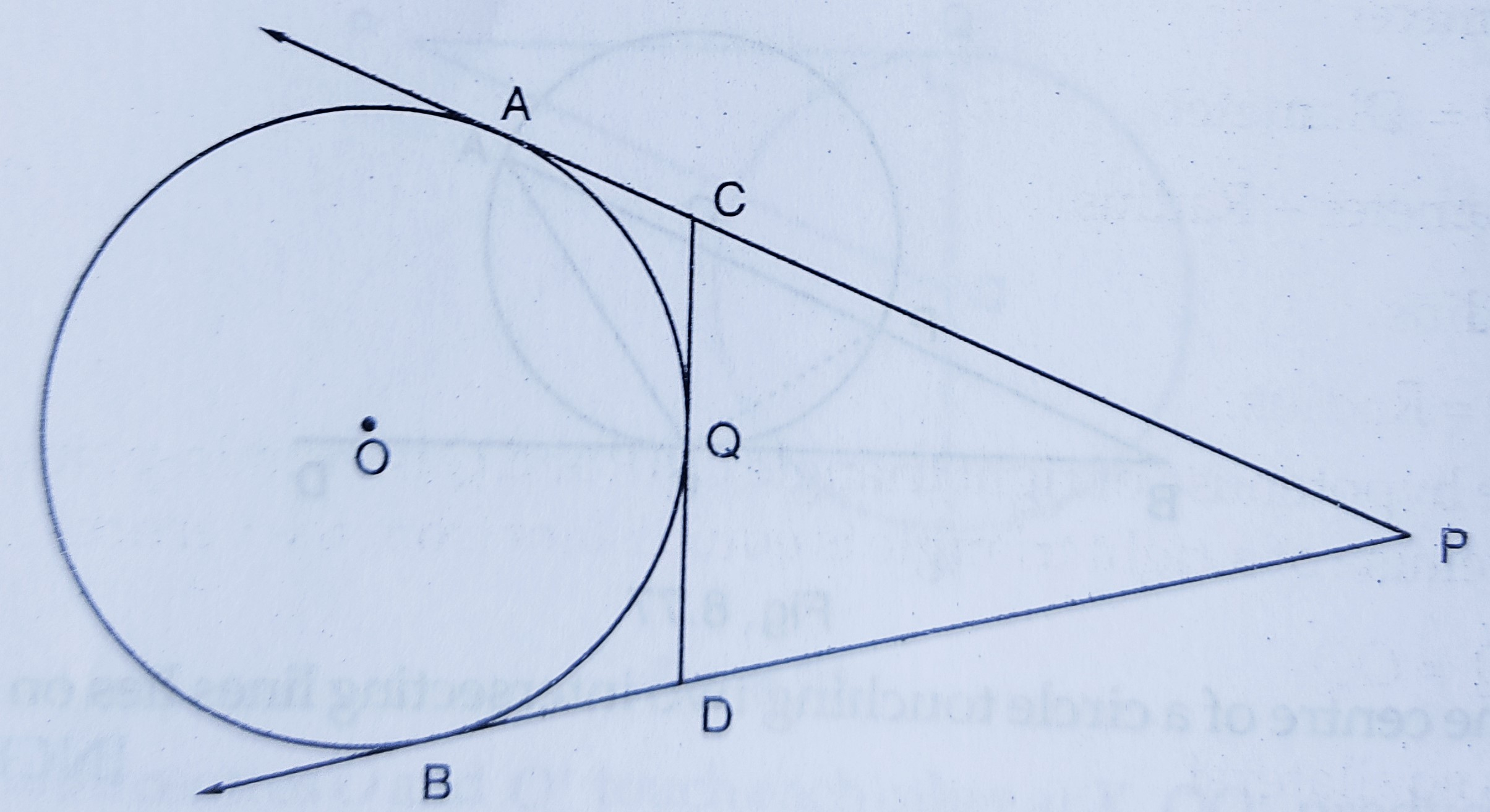# In the figure, $P A$ and $P B$ are tangents to the circle from an external point $P$. $C D$ is another tangent touching the circle at $Q$. If $PA=12\ cm, QC=QD=3\ cm,$ then find $P C+P D$."

Given:

In the figure, $P A$ and $P B$ are tangents to the circle from an external point $P$. $C D$ is another tangent touching the circle at $Q$.

$PA=12\ cm, QC=QD=3\ cm.$

To do:
We have to find $P C+P D$.

Solution:

We know that,

Tangents drawn from an external point are equal.

This implies,

$PA = PB = 12\ cm$.....…(i)

$QC = AC = 3\ cm$......(ii)

$QD = BD = 3\ cm$.......(iii)

Therefore,

$PC + PD = (PA - AC) + (PB - BD)$

$= (12 - 3) + (12 - 3)$    [From (i), (ii) and (iii)]

$= 9 + 9$

$= 18\ cm$

Therefore, $P C+P D = 18\ cm$.